Create specified EAD model object type

## Syntax

``eadModel = fitEADModel(data,ModelType)``
``eadModel = fitEADModel(___,Name,Value)``

## Description

example

````eadModel = fitEADModel(data,ModelType)` creates an exposure at default (EAD) model object specified by `data` and `ModelType`. `fitEADModel` takes in credit data in table form and fits an EAD model. `ModelType` is supported for a `Regression` or `Tobit` model.```

example

````eadModel = fitEADModel(___,Name,Value)` specifies options using one or more name-value pair arguments in addition to the input arguments in the previous syntax. The available optional name-value pair arguments depend on the specified `ModelType`. ```

## Examples

collapse all

This example shows how to use `fitEADModel` to create a `Regression` model for exposure at default (EAD).

```load EADData.mat head(EADData)```
```ans=8×6 table UtilizationRate Age Marriage Limit Drawn EAD _______________ ___ ___________ __________ __________ __________ 0.24359 25 not married 44776 10907 44740 0.96946 44 not married 2.1405e+05 2.0751e+05 40678 0 40 married 1.6581e+05 0 1.6567e+05 0.53242 38 not married 1.7375e+05 92506 1593.5 0.2583 30 not married 26258 6782.5 54.175 0.17039 54 married 1.7357e+05 29575 576.69 0.18586 27 not married 19590 3641 998.49 0.85372 42 not married 2.0712e+05 1.7682e+05 1.6454e+05 ```
```rng('default'); NumObs = height(EADData); c = cvpartition(NumObs,'HoldOut',0.4); TrainingInd = training(c); TestInd = test(c);```

Select Model Type

Select a model type for `Regression` or `Tobit`.

`ModelType ="Regression";`

Select Conversion Measure

Select a conversion measure for the EAD response values.

`ConversionMeasure =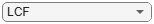"LCF";`

Create `Regression` EAD Model

Use `fitEADModel` to create a `Regression` model using `EADData`.

```eadModel = fitEADModel(EADData,ModelType,'PredictorVars',{'UtilizationRate','Age','Marriage'}, ... 'ConversionMeasure',ConversionMeasure,'DrawnVar','Drawn','LimitVar','Limit','ResponseVar','EAD'); disp(eadModel);```
``` Regression with properties: ConversionTransform: "logit" BoundaryTolerance: 1.0000e-07 ModelID: "Regression" Description: "" UnderlyingModel: [1x1 classreg.regr.CompactLinearModel] PredictorVars: ["UtilizationRate" "Age" "Marriage"] ResponseVar: "EAD" LimitVar: "Limit" DrawnVar: "Drawn" ConversionMeasure: "lcf" ```

Display the underlying model. The underlying model's response variable is the transformation of the EAD response data. Use the `'BoundaryTolerance'`, `'LimitVar'`, and `'DrawnVar'` name-value arguments to modify the transformation.

`disp(eadModel.UnderlyingModel);`
```Compact linear regression model: EAD_lcf_logit ~ 1 + UtilizationRate + Age + Marriage Estimated Coefficients: Estimate SE tStat pValue _________ _________ _______ __________ (Intercept) -2.4745 0.29892 -8.2781 1.6448e-16 UtilizationRate 6.0045 0.19901 30.172 7.703e-182 Age -0.020095 0.0073019 -2.752 0.0059471 Marriage_not married -0.03509 0.13935 -0.2518 0.8012 Number of observations: 4378, Error degrees of freedom: 4374 Root Mean Squared Error: 4.48 R-squared: 0.173, Adjusted R-Squared: 0.173 F-statistic vs. constant model: 305, p-value = 5.7e-180 ```

EAD prediction operates on the underlying compact statistical model and then transforms the predicted values back to the EAD scale. You can specify the `predict` function with different options for the `'ModelLevel'` name-vale argument.

```predictedEAD = predict(eadModel, EADData(TestInd,:), 'ModelLevel', 'ead'); predictedConversion = predict(eadModel, EADData(TestInd,:), 'ModelLevel', 'ConversionMeasure');```

For model validation, use `modelDiscrimination`, `modelDiscriminationPlot`, `modelAccuracy`, and `modelAccuracyPlot`.

Use `modelDiscrimination` and then `modelDiscriminationPlot` to plot the ROC curve.

```ModelLevel =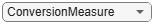"ConversionMeasure"; [DiscMeasure1, DiscData1] = modelDiscrimination(eadModel, EADData(TestInd,:), 'ModelLevel', ModelLevel); modelDiscriminationPlot(eadModel, EADData(TestInd, :), 'ModelLevel', ModelLevel,'SegmentBy','Marriage');```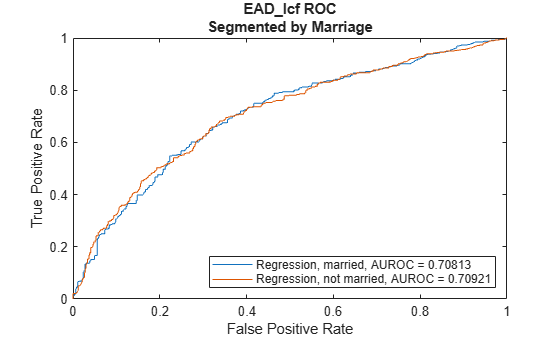Use `modelAccuracy` and then `modelAccuracyPlot` to show a scatter plot of the predictions.

```YData ="Observed"; [AccMeasure1, AccData1] = modelAccuracy(eadModel, EADData(TestInd,:), 'ModelLevel', ModelLevel); modelAccuracyPlot(eadModel, EADData(TestInd,:), 'ModelLevel', ModelLevel, 'YData', YData);```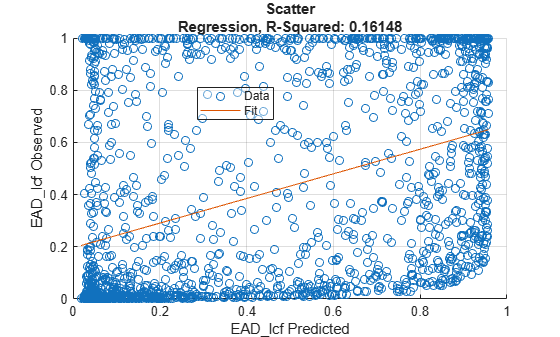Plot a histogram of observed with respect to the predicted EAD.

```figure; histogram(AccData1.Observed); hold on; histogram(AccData1.(('Predicted_' + ModelType))); legend('Observed', 'Predicted');```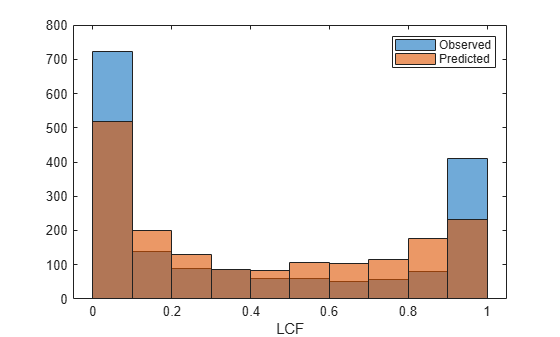## Input Arguments

collapse all

Data for loss given default, specified as a table.

Data Types: `table`

Type of PD model, specified as a scalar string or character vector.

Data Types: `string` | `char`

### Name-Value Arguments

Specify optional comma-separated pairs of `Name,Value` arguments. `Name` is the argument name and `Value` is the corresponding value. `Name` must appear inside quotes. You can specify several name and value pair arguments in any order as `Name1,Value1,...,NameN,ValueN`.

Example: ```eadModel = fitEADModel(EADData,ModelType,'PredictorVars',{'UtilizationRate','Age','Marriage'},'ConversionMeasure',"ccf",'DrawnVar','Drawn','LimitVar','Limit','ResponseVar','EAD')```

The available name-value pair arguments depend on the value you specify for `ModelType`.

Name-Value Pair Arguments for Model Objects

## Output Arguments

collapse all

Loss given default model, returned as an `eadModel` object for a `Regression` or `Tobit` model.

 Baesens, Bart, Daniel Roesch, and Harald Scheule. Credit Risk Analytics: Measurement Techniques, Applications, and Examples in SAS. Wiley, 2016.

 Bellini, Tiziano. IFRS 9 and CECL Credit Risk Modelling and Validation: A Practical Guide with Examples Worked in R and SAS. San Diego, CA: Elsevier, 2019.

 Brown, Iain. Developing Credit Risk Models Using SAS Enterprise Miner and SAS/STAT: Theory and Applications. SAS Institute, 2014.

 Roesch, Daniel and Harald Scheule. Deep Credit Risk. Independently published, 2020.